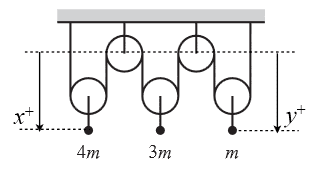# Lagrangian equation for 5 pulley Atwood Machine.

uber_kim

## Homework Statement

Consider the Atwood’s pulley shown below. The masses are 4m, 3m, and m. Let x and y be the directed distances from the centers of the fixed (i.e. inertial) top pulleys for the left and right masses as indicated.http://imgur.com/VXEygxt

a) Write down the Lagrangian for this system in terms of x and y and their time derivatives as usual. (You will need to eliminate z, the distance 3m is from the inertial pulleys using the single rope constraint).

b) By inspection, determine transformations of the form x → x+Kxε, y→y+Kyε that leave the Lagrangian invariant (i.e. determine the generators Kx and Ky). Then use Noether’s theorem to construct the conserved momentum for the system

L=T-U

## The Attempt at a Solution

a) I'm unsure how to deal with the middle pulley. At the moment I have:

T=1/2{4m(x')2 + m(y')2 + 3m(z-(x'))2 + 3m(z-(y'))2}

U=4mgx-mgy+3mg(x-y-z)

b) I don't understand what it's asking. Is there an easier way to explain what it's asking?

Thanks!

## Answers and Replies

Mentor

uber_kim
Oops! Fixed. Thanks.

Reg_S
Did anyone solve this?

•